# Algorithmic problem

The problem of finding a (unique) method (an algorithm) to solve an infinite series of individual problems of the same type. Algorithmic problems arose and were solved in various branches of mathematics throughout its history; however, some of them could not be solved for a long time. The reason for this only became apparent in the 1930s, when an exact definition of an algorithm was given in mathematical logic. It was found that algorithmic problems can be unsolvable, i.e. that the algorithm sought need not exist at all. As a result, the approach to algorithms on the part of mathematicians underwent a radical change, and new algorithmic problems began to be formulated as problems of existence of an algorithm for the solution of a given infinite series of problems of a given type, and of finding such an algorithm if it exists.

Several more exact definitions of algorithm appeared in the theory of algorithms (cf. Algorithms, theory of) practically all at the same time, and they all proved to be essentially equivalent. In each such definition a sufficiently broad class of specific algorithms, closed with respect to the natural operation of combination of algorithms, is singled out. Each statement to the effect that some algorithmic problem is unsolvable is a precise and proved mathematical theorem on the unsolvability of the algorithmic problem under consideration by an algorithm of the given class. In this form these theorems may be regarded as specific, i.e. related to a given class of algorithms. However, all results of this kind may be expressed in terms of the intuitive idea of an algorithm as understood by all mathematicians. This "translation" is based on the so-called Church thesis (depending on the exact specification of the given algorithm, it may also be called Turing's thesis or the normalization principle), which says that the class of algorithms under consideration is universal, i.e. that the essential function of any intuitive algorithm may be performed by algorithms of this class. This thesis is a scientific fact confirmed by the history of mathematics: all algorithms known in mathematics satisfy it and all attempts to construct algorithms not satisfying it have failed. Finally, the thesis is also confirmed by the equivalence of the various proposed definitions of algorithm. Church' thesis cannot be proved, since it deals with the mathematically vague concept of an intuitive algorithm, but it is highly important nevertheless, since it makes it possible to speak of unsolvability of given algorithms in a wide sense, which is generally understood by mathematicians.

The first examples of unsolvable algorithmic problems were noted in the theory of algorithms itself. These include the problem of recognition of belonging to a given non-recursive set of natural numbers, the halting problem of a universal Turing machine, the problem of the recognition of self-applicability of a normal algorithm, etc.

As concerns algorithmic problems outside the theory of algorithms itself, one must mention, first of all, the problem of recognition of validity of formulas of first-order predicate calculus, the unsolvability of which was first proved by A. Church in 1936. Numerous studies of algorithmic problems in model theory are related to these results. Model theory, which deals with non-empty sets with relations defined on them using predicate calculus, was created in the 1930s by A.I. Mal'tsev and A. Tarski. They must also be credited with exact formulations of many algorithmic problems in model theory and with several fundamental results in this field. The elementary theory of a given classof models is the set of all closed formulas of first-order predicate calculus that are true for all models of. The classmay consist of one model. An elementary theoryis solvable or unsolvable, depending on whether or not an algorithm for the recognition of whether or not any given formula belongs to the theoryexists. For a partial review of the methods of studying algorithmic problems in model theory and the results obtained, see . The problem of the solvability of the elementary theory of free groups is the most interesting one mentioned in , and one which has not yet (1977) been solved.

Numerous and varied algorithmic problems also arise during the constructive interpretation of various branches of mathematics. The principal results concerning algorithmic problems in traditional branches of mathematics are given below.

A naturally arising question remained open for a long time: Are unsolvable algorithmic problems specific to the theory of algorithms and mathematical logic? In other words, do there exist unsolvable algorithmic problems in traditional branches of mathematics far removed from mathematical logic? The first result of this kind was obtained in 1949 and 1947 by A.A. Markov and E.L. Post, independently of one another. They proved that the problem of equality (identity) of words for semi-groups — posed by A. Thue as early as 1914 — was unsolvable. In this problem one considers semi-groups defined by a finite number of generators (an alphabet)(1)

and defining relations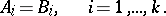(2)

An elementary transformation of the semi-grouphere considered is a transition from the word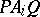to the word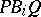or vice versa, where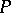and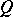are arbitrary words in the alphabet (1). Two wordsand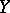in the alphabet (1) are said to be equivalent in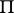if they coincide, or if one can be obtained from the other through a finite sequence of elementary transformations. The set of all equivalence classes with a multiplication operation which is naturally defined by the operation of concatenation of words is just the semi-groupdefined by the generators (1) and relations (2). The problem of word equality (identity) (the word problem) in the semi-groupconsists in finding an algorithm for recognizing if any pair of wordsandofare equivalent inor not, i.e. if the elements ofdefined by them are identical or not. Markov and Post constructed specific semi-groups for which there is no algorithm for solving the equality problem .

The most noteworthy result in this context was obtained by P.S. Novikov , . He showed that the classical word problem in group theory (the equality or identity of words problem) posed by M. Dehn in 1912, which was studied by many experts in algebra throughout the world, was unsolvable. It may be formulated in the same way as the word problem for semi-groups, except that only such systems of relations (2) are considered for which inverse elements for all generators (1) exist in the given semi-group. Novikov also showed that the isomorphism problem, which is very important in group theory — to wit, how to find an algorithm to tell whether or not any two groups are isomorphic — is unsolvable. It was subsequently shown that for any given groupit is impossible to find an algorithm that would tell whether or not an arbitrary group is isomorphic to.

Markov studied the problem of recognition of invariant properties of semi-groups, i.e. of properties that are preserved on passing to isomorphic semi-groups . He showed that if for an invariant semi-group propertythere exists a semi-group with propertyand another semi-group which is not imbeddable in any semi-group with this property, then there is no algorithm by which it is possible to tell whether a given semi-group has the propertyor not. This actually means that almost-all invariant semi-group properties are algorithmically unrecognizable in the class of semi-groups. An analogue of this fundamental theorem has also been obtained in group theory , , . In particular, the conclusion in this case is as follows. Letbe the class of all groups with an invariant property. Two algorithmic problems are related to each such class: the word problem for groups from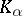, and the problem of recognizing whether or not an arbitrary group belongs to. It was found that for any non-empty classat least one of these algorithmic problems is unsolvable. The same applies to semi-groups as well.

Another problem studied was the specification of the simplest groups and semi-groups in which the word problem is unsolvable. Of the numerous studies on this subject one may mention the proof of the unsolvability of the word problem for the semi-group specified by the following seven simple defining relations :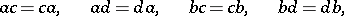At a later date  an example was given of a semi-group with three defining relations and an unsolvable equality problem. No algorithm for solving the word problem in the general case has yet (1977) been found, not even for semi-groups with a single defining relation; it was only constructed for irreducible defining relations . The algorithm solving the word problem for groups with one defining relation dates a long time back , but even for two such relations the question still remains open. The minimum number of defining relations for which examples of groups with unsolvable word problem are known is 12. The problem of word equality for commutative semi-groups has been shown to be solvable. For commutative groups the isomorphism problem has been solved as well. The problem of word equality is solvable for finite groups, for finitely-defined simple groups, for nilpotent groups and for solvable groups of the second order. However, even in the class of solvable groups of the fifth order one can indicate a group given by a corresponding identity of the fifth order and a finite number of defining relations with unsolvable word problem .

The study of the word problem for groups with a limited measure of overlap of the defining words, i.e. with overlap of the left-hand sides of the defining relations, had begun before the unsolvability of the word problem in its general form was demonstrated. The strongest result on this subject at the time of writing (1977) is the proof of the solvability of the word problem in the class of groups defined by systems of defining relations in which the defining words may overlap by less than 1/5 of their length . If the overlap between the words may be as large as 1/3 of their length, an example of a group with unsolvable word problem becomes quite simple. In the range between 1/5 and 1/3 the problem remains open. Similar studies were also conducted for semi-groups. It was found that for semi-groups with maximum measure of overlap of the defining words less than 1/2 the word problem is solvable, but examples are known of semi-groups with an unsolvable word problem in which the maximum overlap of the defining words is 1/2.

The most prominent topological algorithmic problem is the classical decision problem for homeomorphism of-dimensional manifolds. Markov constructed an example of a four-dimensional manifoldfor which it is impossible to construct an algorithm to tell whether or not an arbitrary four-dimensional manifold is homeomorphic to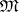. It is known that forthe solution of this problem is positive; for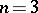the problem remains open; for four-dimensional and higher-dimensional manifolds the problems of recognition of combinatorial and homotopy equivalence of manifolds are also unsolvable. One may also mention that ifis a polyhedron for which the word identity problem of its fundamental group is unsolvable, no algorithm can be constructed forthat recognizes the bounded homotopy (or the free homotopy) of two arbitrary paths onpassing through a common point. A positive solution was obtained for the problem of conjugation in braid groups; this problem is equivalent to the topological problem of recognizing the equivalence of braids .

One of the most famous algorithmic problems in mathematics is Hilbert's 10th problem: To find an algorithm by which to tell whether or not a system of Diophantine equations with integer coefficients has a solution in integers. After many examples of unsolvable algorithmic problems had become known, it was suggested that this problem was unsolvable as well. Moreover, a team of American mathematicians succeeded in proving that the unsolvability of Hilbert's tenth problem follows from the existence of a two-place Diophantine predicate of exponential growth , . However, they were unsuccessful in constructing such a predicate; they merely demonstrated that the recognition of the existence of integer solutions of exponential equations was an unsolvable problem. The desired Diophantine predicate of exponential growth was first constructed in 1970 , ; this was the first proof ever given of the unsolvability of Hilbert's tenth problem.

In the theory of algorithms the existence of unsolvable algorithmic problems of various degrees of unsolvability was proved. Many studies of algorithmic problems arising in mathematics showed that each such problem had, in the general case, a maximum degree of unsolvability, while their special instances (e.g. the word identity problem in specific semi-groups or groups) may have any pre-given degree of unsolvability.